### IMO Shortlist 1988 problem 18

Kvaliteta:
Avg: 0,0
Težina:
Avg: 0,0
Consider 2 concentric circle radii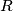$R$ and$r$ (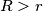$R > r$) with centre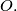$O.$ Fix$P$ on the small circle and consider the variable chord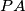$PA$ of the small circle. Points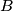$B$ and$C$ lie on the large circle;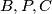$B,P,C$ are collinear and$BC$ is perpendicular to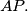$AP.$

i.) For which values of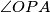$\angle OPA$ is the sum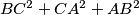$BC^2 + CA^2 + AB^2$ extremal?

ii.) What are the possible positions of the midpoints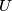$U$ of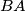$BA$ and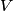$V$ of$AC$ as$\angle OPA$ varies?
Izvor: Međunarodna matematička olimpijada, shortlist 1988